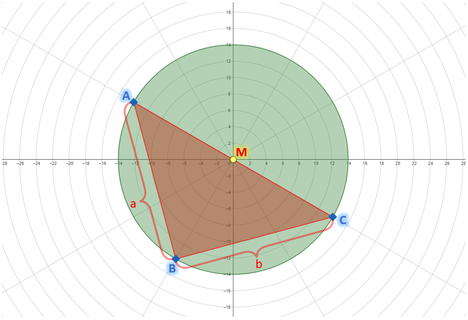# In Search of Prometheussiamsplash.5DIU Take-Off Programming...57/60/98
Limits 1s, 32 MB

A ship named Prometheus disappeared in the Atlantic Ocean for unknown reasons. Not a single clue was even found about the ship. This is not the first time, five more ships from different countries have also disappeared in the same region before.

ACM (Artificially Correct Mathematicians) are known for their exclusive service using Artificial Intelligence. They don’t do any physical operations. Instead, they use special devices powered by AI. They are currently investigating the ship disappearances.

To investigate, they have identified a triangular area (marked red) in the ocean where there is a high probability of finding Prometheus.To get more precise information, they have placed three beacons $A$, $B$ and $C$ (marked blue) on the triangle’s vertices.

After that, they placed their AI radar from space exactly at the point $M$ (marked yellow), which will inform them about anomalies in the circular area (marked green) that falls in its search radius.

Note:

• Point $M$ is the midpoint of $AC$.

• $A$, $B$ and $C$ always will be on the border of the radar’s range.

• It is guaranteed that $\angle ABC$ always $90\degree$.

• The anomalies only occur within the triangular area covered by beacons $A$, $B$, and $C$.

However, the AI radar is not only monitoring the triangular area but also the entire circular area within its radius. So, if the above picture and the notes are observed it can be seen that $AC$ is the diameter of the radar’s range.

ACM has limited funds for this task. So they want to cut costs and find alternatives. Before approval, the corporation wants to know how much area is monitored where no anomalies will occur (marked green).

Now, you are given $a$, $b$ which are the distance between beacons $A$ and $B$, and $B$ and $C$ respectively. Find the total area that is monitored where no anomalies will occur (marked green).

Some important formulas to be noted:

• Area of a right-angled triangle =  $½ \times base \times height$.

• For a right-angled triangle, $hypotenuse^2 = base^2 + height^2$.

• Area of a circle = $π \times r^2$.

• Use $π = 3.1416$ for this problem.

## Input

The input will contain two positive integers $a$ and $b$ $(1 \le a, b \le 100)$ — denoting the distance between beacons $A$ and $B$, and $B$ and $C$ respectively.

## Output

Print the total area that is monitored where no anomalies will occur (marked green). Print $6$ digits after the decimal point.

## Samples

InputOutput
4 7

37.051000

InputOutput
100 100

10708.000000


Use double data type for this problem.

### Submit